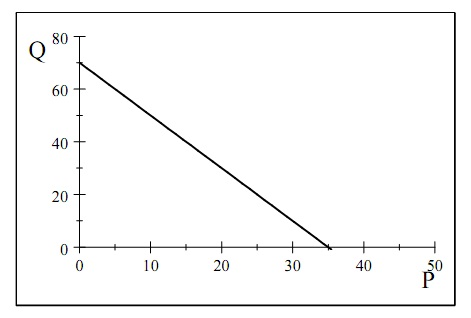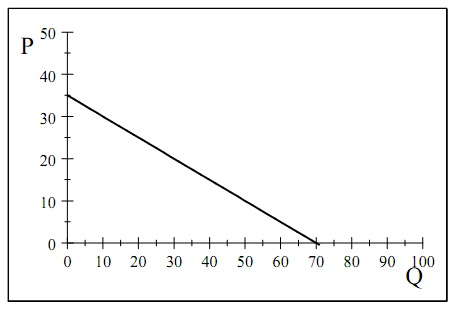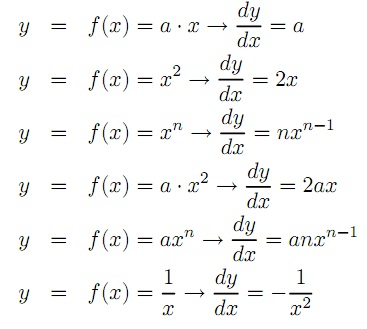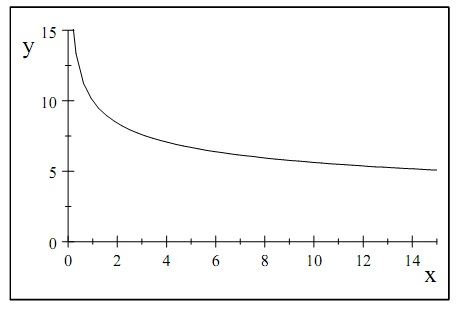#### Example for demand function

Example for demand function:

Let consider an example, consider the following demand function for beer:

QD = 100 − 2. p + 0.05.ps − 0.25.pc − 0.00125 .Y

QD is here expressed in a volume measure (liters or bottles or cans etc) per month and prices and income in money (kr, €, \$, £ etc). The substitute good maybe wine and the complement good sausage. If p = 10kr/bottle, ps = 50kr/bottle, pc = 30kr/kilo and Y = 20000kr/month. Inserting these numbers:

QD = 100 – 2x10 + 0.05x50 − 0.25x30 − 0.00125x20000 = 50,

i.e., this household, or consumer, demands 50liters of beer per month. Now, the demand curve is a graph which shows the relationship between the own price and quantity demanded, so if we aggregate all the numbers above except for the price we get:

Q = (100 + 0.05 x50 − 0.25x30 − 0.00125x20000) – 2xp

Q = 70 – 2.p

This demand curve is plotted in figure shown below, but note that here we have the price (variable x) on the horizontal axis.If we instead want to express price as a function of quantity we have to solve for the inverse demand curve:

p (Q) = 35 – 1/2.QThe slope of the (direct) demand curve shows the marginal effect of the increase in the own price (holding all other factors constant) on quantity demanded. In the case with a linear demand curve it is OK to use the ratio of two “big” changes:
?Q/?p = αo,

to measure the marginal effect, but if the demand curve is non-linear its slope changes as we slide down the curve. In this case it is more appropriate to consider the ratio of two very small (or “in?nitesimal”) changes, such a ratio is called a derivative of a function and it is denoted as:

∂Q/∂p = αo (p)

The coefficient αo is no longer a constant, but varies along the demand curve (with Q, which in turn depends on p). There are some basic rules of derivatives which are practical to know, the most important ones are (for a general function y = f(x)):An important example of a non-linear demand function is the following:

Q = Ap, ε > 0

The derivative of this function is:

∂Q/∂p = -εAp-ε-1

For example, if A = 10, p = 2 and ε = 1/4, the quantity demanded is equal to: Q = 10. 2-1/4 = 8.41 and the derivative at this point on the demand curve is:

∂Q/∂p = -1/4.10 .2-1/4-1 = -1.051. This shows that a unit increase in price will decrease quantity demanded by 1.05 units, approximately. This demand function is illustrated in figure shown below:Latest technology based Economics Online Tutoring Assistance

Tutors, at the www.tutorsglobe.com, take pledge to provide full satisfaction and assurance in Intermediate Microeconomics homework help via online tutoring. Students are getting 100% satisfaction by online tutors across the globe. Here you can get homework help for Intermediate Microeconomics, project ideas and tutorials. We provide email based Intermediate Microeconomics homework help. You can join us to ask queries 24x7 with live, experienced and qualified online tutors specialized in Intermediate Microeconomics. Through Online Tutoring, you would be able to complete your homework or assignments at your home. Tutors at the TutorsGlobe are committed to provide the best quality online tutoring assistance for Economics homework help and assignment help services. They use their experience, as they have solved thousands of the Economics assignments, which may help you to solve your complex issues of Intermediate Microeconomics. TutorsGlobe assure for the best quality compliance to your homework. Compromise with quality is not in our dictionary. If we feel that we are not able to provide the homework help as per the deadline or given instruction by the student, we refund the money of the student without any delay.